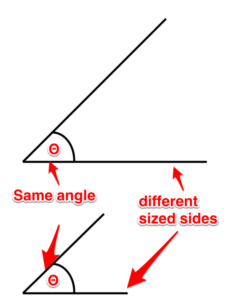# 1.1: Angles

$$\newcommand{\vecs}{\overset { \rightharpoonup} {\mathbf{#1}} }$$ $$\newcommand{\vecd}{\overset{-\!-\!\rightharpoonup}{\vphantom{a}\smash {#1}}}$$$$\newcommand{\id}{\mathrm{id}}$$ $$\newcommand{\Span}{\mathrm{span}}$$ $$\newcommand{\kernel}{\mathrm{null}\,}$$ $$\newcommand{\range}{\mathrm{range}\,}$$ $$\newcommand{\RealPart}{\mathrm{Re}}$$ $$\newcommand{\ImaginaryPart}{\mathrm{Im}}$$ $$\newcommand{\Argument}{\mathrm{Arg}}$$ $$\newcommand{\norm}{\| #1 \|}$$ $$\newcommand{\inner}{\langle #1, #2 \rangle}$$ $$\newcommand{\Span}{\mathrm{span}}$$ $$\newcommand{\id}{\mathrm{id}}$$ $$\newcommand{\Span}{\mathrm{span}}$$ $$\newcommand{\kernel}{\mathrm{null}\,}$$ $$\newcommand{\range}{\mathrm{range}\,}$$ $$\newcommand{\RealPart}{\mathrm{Re}}$$ $$\newcommand{\ImaginaryPart}{\mathrm{Im}}$$ $$\newcommand{\Argument}{\mathrm{Arg}}$$ $$\newcommand{\norm}{\| #1 \|}$$ $$\newcommand{\inner}{\langle #1, #2 \rangle}$$ $$\newcommand{\Span}{\mathrm{span}}$$$$\newcommand{\AA}{\unicode[.8,0]{x212B}}$$

## What’s the deal with angles anyway?

Before we even get into trigonometry, we need to discuss angles. Don’t worry. Things are not going to get too crazy. I promise. Let’s go over the basics first.

Degree. One-three-hundred-and-sixtieth of the circumference of a circle. It is also the unit by which we measure angles.Figure 1. Degrees

Angle. This is the space between two intersecting lines.Figure 2. Angle

Complementary angles. These are two angles whose sum equals 90 degrees.Figure 3. Complementary angle

Supplementary angles. These are two angles whose sum equals 180 degrees.Figure 4. Supplementary angle

Acute angle. An angle that is less than 90 degrees.Figure 5. Acute angle

Obtuse angle. An angle that is greater than 90 degrees.Figure 6. Obtuse angle

Similar angles. It is possible for triangles to each have different sized sides but share the same sized angles. These are called similar angles.Figure 7. Similar angles

Right angle. This is an angle that is 90 degrees.Figure 8. Right angle

There is a ton of information about angles that we don’t need to get into. Remember: Try not to overcomplicate things. Just focus on the basics and you’ll be fine.

This page titled 1.1: Angles is shared under a CC BY 4.0 license and was authored, remixed, and/or curated by Chad Flinn (BC Campus) via source content that was edited to the style and standards of the LibreTexts platform; a detailed edit history is available upon request.